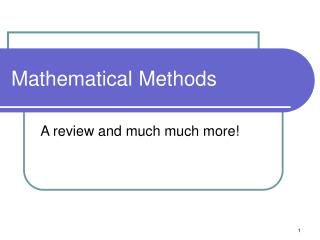Download PresentationMathematical Methods

# Mathematical Methods

Download Presentation## Mathematical Methods

- - - - - - - - - - - - - - - - - - - - - - - - - - - E N D - - - - - - - - - - - - - - - - - - - - - - - - - - -
##### Presentation Transcript

1. Mathematical Methods A review and much much more!

2. Trigonometry Review • First, recall the Pythagorean theorem for a 900 right triangle • a2+b2 = c2 c b a

3. Trigonometry Review • Next, recall the definitions for sine and cosine of the angle q. • sin q = b/c or • sin q = opposite / hypotenuse • cos q = b/c • cos q = adjacent / hypotenuse • tan q = b/a • tan q = opposite / adjacent c b q a

4. Trigonometry Review • Now define in general terms: • x =horizontal direction • y = vertical direction • sin q = y/r or • sin q = opposite / hypotenuse • cos q = x/r • cos q = adjacent / hypotenuse • tan q = y/x • tan q = opposite / adjacent r y q x

5. r x q y Rotated • If I rotate the shape, the basic relations stay the same but variables change • x =horizontal direction • y = vertical direction • sin q = x/r or • sin q = opposite / hypotenuse • cos q = y/r • cos q = adjacent / hypotenuse • tan q = x/y • tan q = opposite / adjacent

6. r y q x Unit Circle I • Now, r can represent the radius of a circle and q, the angle that r makes with the x-axis • From this, we can transform from ”Cartesian” (x-y) coordinates to plane-polar coordinates (r-q) II IV III

7. The slope of a straight line • A non-vertical has the form of • y = mx +b • Where • m = slope • b = y-intercept • Slopes can be positive or negative • Defined from whether y = positive or negative when x >0 Positive slope Negative slope

8. x2 , y2 x1 , y1 Definition of slope

9. The Slope of a Circle • The four points picked on the circle each have a different slope. • The slope is determined by drawing a line perpendicular to the surface of the circle • Then a line which is perpendicular to the first line and parallel to the surface is drawn. It is called the tangent

10. The Slope of a Circle • Thus a circle is a near-infinite set of sloped lines.

11. The Slope of a Curve • This is not true for just circles but any function! • In this we have a function, f(x), and x, a variable • We now define the derivative of f(x) to be a function which describes the slope of f(x) at an point x • Derivative = f’(x) f’(x) f(x)

12. Differentiating a straight line • f(x)= mx +b • So • f’(x)=m • The derivative of a straight line is a constant • What if f(x)=b (or the function is constant?) • Slope =0 so f’(x)=0

13. Power rule • f(x)=axn • The derivative is : • f’(x) = a*n*xn-1 • A tricky example:

14. Differential Operator • For x, the operation of differentiation is defined by a differential operator • And the last example is formally given by

15. 3 rules • Constant-Multiple rule • Sum rule • General power rule

16. 3 Examples Differentiate the following:

17. Functions • In mathematics, we often define y as some function of x i.e. y=f(x) • In this class, we will be more specific • x will define a horizontal distance • y will define a direction perpendicular to x (could be vertical) • Both x and y will found to be functions of time, t • x=f(t) and y=f(t)

18. Derivatives of time • Any derivative of a function with respect to time is equivalent to finding the rate at which that function changes with time

19. Can I take the derivative of a derivative? And then take its derivative? • Yep! Look at Called “2nd derivative” 3rd derivative

20. Can I reverse the process? • By reversing, can we take a derivative and find the function from which it is differentiated? • In other words go from f’(x) → f(x)? • This process has two names: • “anti-differentiation” • “integration”

21. Why is it called integration? • Because I am summing all the slopes (integrating them) into a single function. • Just like there is a special differential operator, there is a special integral operator: Called an “indefinite integral” 18th Century symbol for “s” Which is now called an integral sign!

22. What is the “dx”? • The “dx” comes from the differential operator • I “multiply” both sides by “dx” • The quantity d(f(x)) represents a finite number of small pieces of f(x) and I use the “funky s” symbol to integrate them • I also perform the same operation on the right side

23. Constant of integration • Two different functions can have the same derivative. Consider • f(x)=x4 + 5 • f(x)=x4 + 6 • f’(x)=4x • So without any extra information we must write • Where C is a constant. • We need more information to find C

24. Power rule for integration

25. Examples

26. Definite Integral • The definite integral of f’(x) from x=a to x=b defines the area under the curve evaluated from x=a to x=b f(x) x=a x=b

27. Mathematically Note: Technically speaking the integral is equal to f(x)+c and so (f(b)+c)-(f(a)+c)=f(b)-f(a)

28. What to practice on: • Be able to differentiate using the 4 rules herein • Be able to integrate using power rule herein